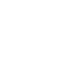in-the-figure-ac-10cm-pc-15cm-pq-12-cm-find-pb-6-cm7-cm8-11Top matches
Q1.
In the figure, AC = 10cm, PC = 15cm, PQ = 12 cm, then PB = __ cm .Q2.

In the figure, AC = 10cm, PC = 15cm, PQ = 12 cm, find PB.Q3.

Question: In the figure, AC = 10cm, PC = 15cm, PQ = 12 cm, find PB

Q4.

In the figure, AQ = 1 cm, PC = 15cm, PQ = 12 cm and AB = 6 cm, find CB.Q5.

In the figure, AC = 10 cm, AB = 8 cm, PC = 15 cm, then PB = __ cm .